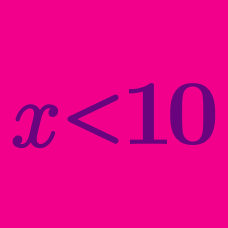Algebra

# Linear Inequalities - Problem Solving

If $$x+y = 7$$ and $$4 \leq x \leq 19,$$ what is the range of $$y?$$

If the inequality $$a^2(x+1)>576 x+24a$$ holds for all real numbers $$x,$$ what is the constant $$a?$$

Let $$x>\frac{14}{13}$$ be the solution to the inequality $$bx-(a+b)<0,$$ where $$a$$ and $$b$$ are constants. Then what is the solution to the inequality $$ax+2a+b<0?$$

A factory produces two products $$A$$ and $$B$$ using raw materials $$p$$ and $$q$$. It uses $$3$$ units of $$p$$ and $$1$$ unit of $$q$$ to produce one unit of $$A$$. It uses $$1$$ unit of $$p$$ and $$2$$ units of $$q$$ to produce one unit of $$B$$. The maximum daily supplies are $$30$$ units of $$p$$ and $$20$$ units of $$q.$$ If the profit per unit of $$A$$ is $$\3$$ and the profit per unit of $$B$$ is $$\2$$, what is the maximum total daily profit (in dollars)?

Real numbers $$x$$ and $$y$$ satisfy $$-3 < x < 1$$ and $$-1 < y < 5$$. What is the range of $$x - y ?$$

×